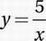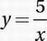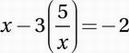# SAT Math Multiple Choice Question 630: Answer and Explanation

### Test Information

Question: 630

15. x - 3y = -2The values of x that satisfy the system of equations above also satisfy which of the following equations?

• A. (x - 5)(x + 3) = 0
• B. (x - 3)(x + 5) = 0
• C. (x - 2)(x - 5) = 0
• D. (x + 2)(x + 5) = 0

Explanation:

B

Advanced Mathematics (linear and nonlinear systems) HARD

First, notice that the question is only asking us to find values of x, so it's a good idea to substitute in order to eliminate y from the system.

x - 3y = -2

Substitute:Multiply by x and simplify:

x2 - 15 = -2x